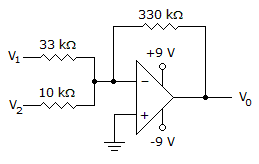# Electronic Devices - Op-Amp Applications - Discussion

Discussion Forum : Op-Amp Applications - General Questions (Q.No. 2)
2.
Calculate the output voltage if V1 = –0.2 V and V2 = 0 V.0 V
–6.6 V
–4 V
2 V
Explanation:
No answer description is available. Let's discuss.
Discussion:
13 comments Page 1 of 2.

Amit said:   6 years ago
As we see in the circuit, -o.2 is applied at the negative terminal so the op-amp is inverting op-amp. By general description, we calculate drop across 33k by ohms law. We get 1/165 amp and in other branches we applied same ohms law and we get 0 amp coz v=0v. Now add both the currents and applied again ohms law at feedback branch 330k we get vo= 2volt.

Muhammad Younas said:   7 years ago
Good work, Thanks for explaining it.
(1)

Neeharika said:   7 years ago
By nodal analysis (0 -V1)/33k+(0-V0)/330k = 0.
0.2/33k-V0/330k = 0.
V0 = 2v.
(1)

Raviraj said:   7 years ago
vo = -[330/33 (-0.2)] = 2v.
(1)

Surya said:   7 years ago
If the voltage source is connected to the positive terminal of an op amp and ground is connected to the negative terminal of the op amp then it's called as "non-inverting amplifier". If the terminals are reversed then acts as inverting amplifier.
(1)

Seema Rajput said:   8 years ago
Vo = (-Rf/R1)V1 + (-Rf/R2)V2.
= (-330k/33k) * (- 0.2v) + (- 330/10k) * 0.
= (- 10) * (-0.2) + 0.
Vo = 2V.
(5)

Mb3r said:   8 years ago
What the different between non and inverting op amps?

Indu said:   9 years ago
Here given the amplifier is summing opamp and the solution of the given problem.

vo = -[330/33 (-0.2)] = 2 v.

MMA said:   10 years ago
Vout = -330k{(-0.2/33k)+(0/10k)}.

= -330k(-0.2/33k).

= 2 volt.